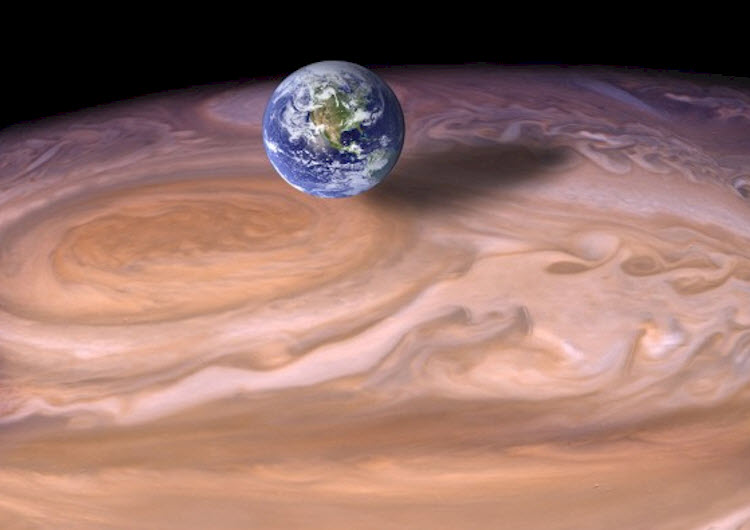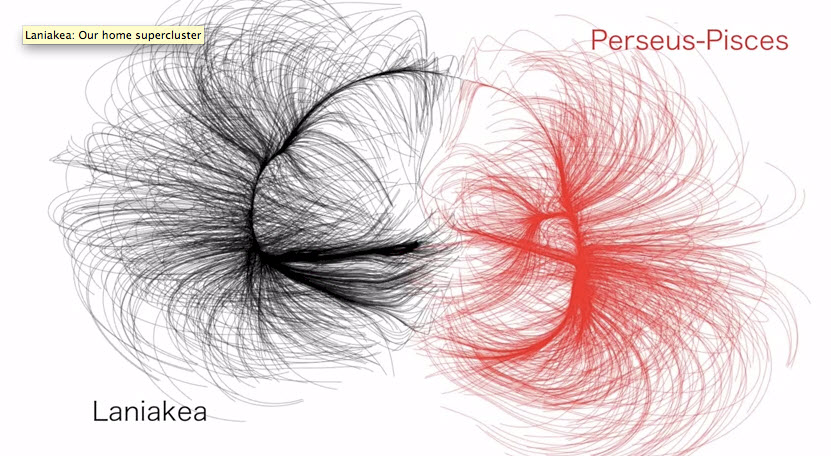Notes on video lecture:
Orders of Magnitude
Notes taken by Edward Tanguay on December 19, 2015 (go to class or lectures)Choose from these words to fill the blanks below:
compact, planets, car, stars, money, dwarf, universe, super, restaurant, apartment, clusters, light, vacation, dollar, planetary
orders of magnitude in terms of
1. a
10^0 dollars
one Snickers bar
2. ten dollars
10^1 dollars
a                disc
3. one hundred dollars
10^2 dollars
dinner at a nice
4. one thousand dollars
10^3 dollars
a nice
5. ten thousand dollars
10^4 dollars
a new
6. one hundred thousand dollars
10^5 dollars
an                    in Paris
eight orders of magnitude / size scales
1. human being
10^0 meters
2.
next step up in structure
range from 10^6 - 10^7 meters
the jump in orders of magnitude between the size of a man and the size of a planet is larger than the different between the price of a candy bar and a nice apartment in Paris
3. stars
10^8 - 10^12 meters
stars to giant stars
4.                    sytems
our solar system
10^13 meters
5. galaxies
vast collections of
10^19 meters
a jump of nine orders of magnitude, more than from man to planet
6. galaxy
groups of galaxies
10^22 meters
7.            clusters
clusters of clusters of galaxies
10^24 meters
8. size of
the observable universe, i.e. the bubble of            which encompasses the universe which we can see
but there is most likely more universe beyond even that
our understand now is the the universe is, in fact, infinite, on the largest scales

## Ideas and Concepts:

A sense of perspective via this tonight's Modern Astronomy class: "Regarding the size of objects in the universe from the size of a human being and upward, we typically consider eight orders of magnitude:(1) HUMAN BEINGS we can say is on average about 1 meter high or 10^0 meters, the (2) PLANETS are the next step up in size structure and range from 10^6 - 10^7 meters, next we have (3) STARS which range from dwarf stars to giant stars and range from a size of 10^8 - 10^12 meters, then (4) PLANETARY SYSTEMS, e.g. our solar system have a size of around 10^13 meters, then come (5) GALAXIES which are vast collections of stars which are 10^19 meters, which, notice is a jump of nine orders of magnitude, more than from the size of man to the size of planets, then come (6) GALAXY CLUSTERS, or groups of galaxies, which are 10^22 meters, then (7) SUPERCLUSTERS, or clusters of clusters of galaxies which are 10^24 meters, such as as our home supercluster Laniakea, and finally we have the size of (8)THE UNIVERSE itself, which we often consider to be merely the observable universe, i.e. the bubble of light which encompasses the universe which we can see, but since there is most likely much more universe beyond even that, our current understanding of the universe is that it is, in fact, infinite on the largest scales."